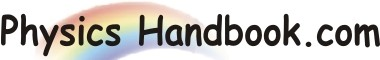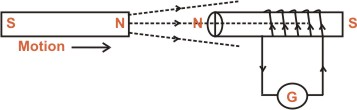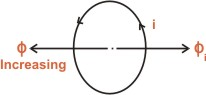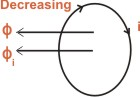HOME TOPICS DEFINITIONS TABLES LAWS INVENTIONS EXPERIMENTS QUIZ VIDEOS
 Lenz's Law Emil Lenz gave the following simple rule to find the direction of induced current: The induced current will flow in such a direction so as to oppose the cause that produces it.Let us apply Lenz's law to figure given above. Here the N-pole of the magnet is approaching a coil of several turns. As the N-pole of the magnet moves towards coil, the magnetic flux linking the coil increases. Therefore, an e.m.f and hence current is induced in the coil according to faraday's laws of electromagnetic induction. According to Lenz's law, the direction of the induced current will be such so as to oppose the cause that produces it. In the present case, the cause of the induced current is the increasing magnetic flux linking the coil. Therefore, the induced current will set up magnetic flux that opposes the increase in flux through the coil. Therefore, the induced current will set up magnetic flux that opposes the increase in flux through the coil. This is possible only if the left hand face of the coil becomes N-pole. Once we know the magnetic polarity of the coil face, the direction of the induced current can be easily determined by applying right hand rule for the coil. The Lenz's law can be summed up as under: If the magnetic flux Ф linking a coil will flow in such a direction so as to oppose the increase in flux i.e. the induced current will produce flux as shown in figure given below,If magnetic flux Ф linking a coil is decreasing, the induced current i in the coil will flow in such a direction so as to oppose the decrease in the flux i.e. the induced current will produce flux Ф to aid the flux Ф as shown in figure given below.